NCERT Solutions for Class 6 Maths Chapter 13 Symmetry Ex 13.2 are part of NCERT Solutions for Class 6 Maths. Here we have given NCERT Solutions for Class 6 Maths Chapter 13 Symmetry Ex 13.2.

 Board CBSE Textbook NCERT Class Class 6 Subject Maths Chapter Chapter 13 Chapter Name Symmetry Exercise Ex 13.2 Number of Questions Solved 8 Category NCERT Solutions

## NCERT Solutions for Class 6 Maths Chapter 13 Symmetry Ex 13.2

Question 1.
Find the number of lines of symmetry for each of the following shapes :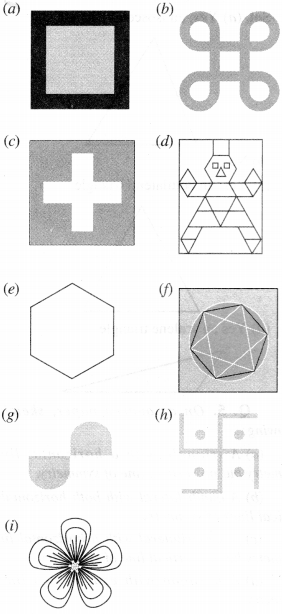Solution :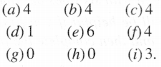Question 2.
Copy the triangle in each of the following figures, on squared paper. In each case, draw the line(s) of symmetry if any and identify the type of triangle. (Some of you may like to trace the figures and try paper-folding first!)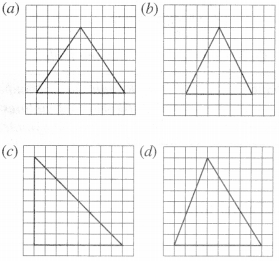Solution :Question 3.
Complete the following table :Solution :Question 4.
Can you draw a triangle which has
(a) exactly one line of symmetry?
(b) exactly two lines of symmetry?
(c) exactly three lines of symmetry?
(d) no lines of symmetry?
Sketch a rough figure in each case.
Solution :
(a) Yes; an isosceles triangle(b) No
(c) Yes; an equilateral triangle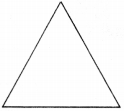(d) Yes, an equilateral triangle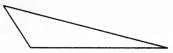Question 5.
On a squared paper, sketch the following:
(a) A triangle with a horizontal line of symmetry’ but no vertical line of symmetry.
(b) A quadrilateral with both horizontal and vertical lines of symmetry.
(c) A quadrilateral with a horizontal line of symmetry but no vertical line of symmetry.
(d) A hexagon with exactly two lines of symmetry.
(e) A hexagon with six lines of symmetry.
(Hint: It will be helpful if you first draw the lines of symmetry and then complete the figures.)
Solution :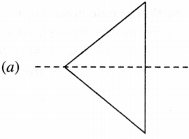Question 6.
Trace each figure and draw the lines of symmetry, if any :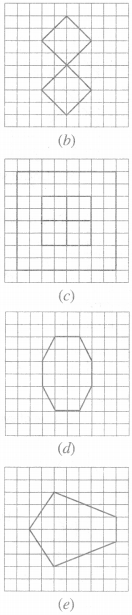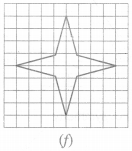Solution :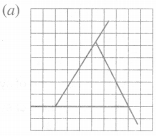no line symmetry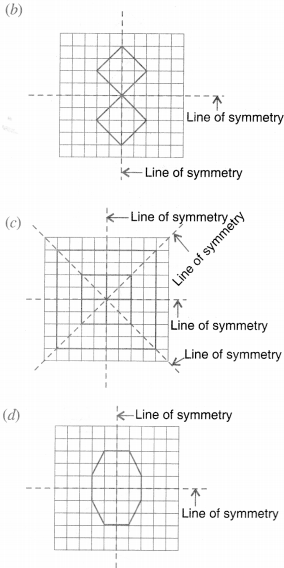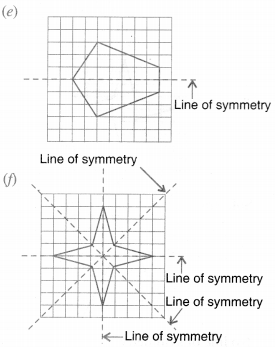Question 7.
Consider the letters of English alphabets A to Z. List among them the letters which have
(a) vertical lines of symmetry (like A)
(b) horizontal lines of symmetry (like B)
(c) no lines of symmetry (like Q)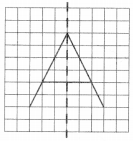Solution :
(a) A, H, I, M, O, T, U, V, W, X, Y
(b) B, C, D, E, H, I, K, O, X
(c) F, G, J, L, N, P, Q, R, S, Z

Question 8.
Given here are figures of a few folded sheets and designs drawn about the fold. In each case, draw a rough diagram of the complete figure that would be seen when the design is cut off.Solution :We hope the NCERT Solutions for Class 6 Maths Chapter 13 Symmetry Ex 13.2 help you. If you have any query regarding NCERT Solutions for Class 6 Maths Chapter 13 Symmetry Ex 13.2, drop a comment below and we will get back to you at the earliest.